Technical Article

# Electric Motor Sizing and Selection Explained

November 15, 2022 by Simon Mugo

## The article guides electrical engineers on electric motor sizing and selection to satisfy their power system requirements.

For an electric power system to work well, the secret lies in the proper sizing and selection of important components.  Sizing and properly selecting an electric motor goes a long way to improve reliability and performance while making equipment cost-effective. This article will guide you through selecting and sizing an electric motor.

### Determining the Motor Drive Mechanism

The first step is determining the motor drive mechanism. The drive mechanism includes a belt drive, shaft, direct rotation, pulley, ball screw or rack, and pinion.

While determining the drive mechanism, also decide the dimensions, which are important for motor sizing:

• Each part’s dimension and mass
• Coefficient of friction of each moving part sliding surface

The next step is determining the motor’s parameters and specifications, including:

• Operating time and speed
• Positioning distance and time
• Resolution
• Stopping accuracy
• Voltage and power supply
• Position holding
• Environment of operation
• Specific requirements and features like closed loop, open loop, feedback, programmable, agent approval, and IP rating

To determine motor performance, establish the following three factors:

• Motor speed
• Motor torque
• Moment of inertia

Once the above three factors are calculated, the motor will be selected depending on the values obtained for speed, inertia, and torque. A range of of motors exists to choose from, such as servo, AC, stepper, and brushless motors.

### Sizing the Motor: Calculations

For successful motor sizing, three important factors must be determined: torque, inertia, and speed.

### Determining the Moment of Inertia

Moment of inertia is the measure of any given object’s resistance to its rotation rate changes. An object at rest has a zero moment of inertia.

Trying to move an object focuses on changing its speed from point zero to any level. This is accompanied by the change in the moment of inertia.

#### The Fundamental Equation of Inertia(J)##### Figure 1. Fundamental equation of inertia diagram. Image used courtesy of Simon Mugo

$J=\smallint\smallint\smallint \rho(x,y,z)(x^{2}+y^{2})dxdydz$

#### Rotating Object Moment of Inertia Calculation

J = mL2

Where

m = mass

L = The distance between the center of rotation and the center of gravity.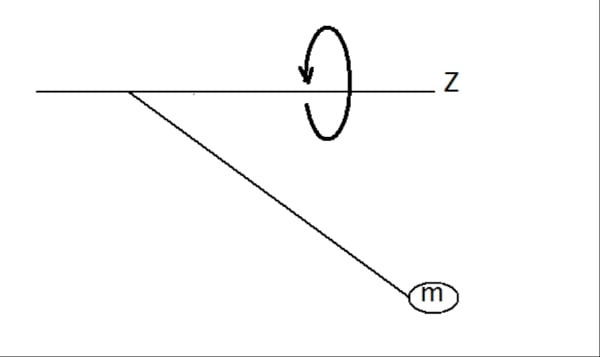#### Calculating the Cylinder’s Moment of Inertia##### Figure 3. Solid cylinder. Image used courtesy of Simon Mugo

From Figure 3, the formula for calculating the moment of inertia for the solid cylinder can be derived as:

$J_{x}=\frac{1}{8}mD_{1}^{\,\,\,2}=\frac{\pi}{32}\rho LD_{1}^{\,\,\,4}$

And

$J_{y}=\frac{1}{4}m(\frac{D_{1}^{\,\,\,2}}{4}+\frac{L^{2}}{3})$

Where

Jx= inertia along x - axis

Jy = Inertia along y - axis

m = mass of the cylinder

D1 = The Cylinder Outer Diameter

ρ = Density of the Cylinder

L = Height of the Cylinder

#### Calculating the Hollow Cylinder Moment of Inertia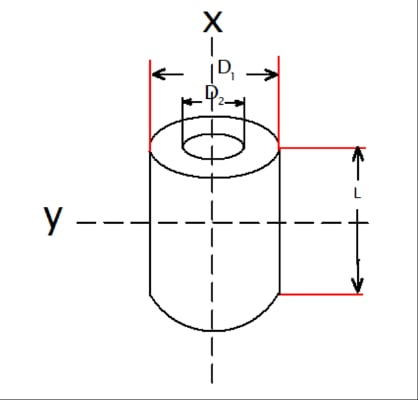##### Figure 4. Hollow cylinder. Image used courtesy of Simon Mugo

From Figure 4, the formula for calculating the moment of inertia for the hollow cylinder which leads to the leading nations can be derived as:

$J_{x}=\frac{1}{8}m\Big(D_{1}^{\,\,\,2}-D_{2}^{\,\,\,2}\Big)=\frac{\pi}{32}\rho L(D_{1}^{\,\,\,2}-D_{2}^{\,\,\,4})$

And

$J_{y}=\frac{1}{4}m(\frac{D_{1}^{\,\,\,2}-D_{2}^{\,\,\,2}}{4}+\frac{L^{2}}{3})$

Where

Jx = inertia along x - axis

Jy = Inertia along y - axis

m = mass of the Hollow cylinder

D1 = The Hollow Cylinder Outer Diameter

D2 = The Hollow Cylinder inner diameter

ρ = Density of the Hollow Cylinder

L = Height of the Hollow Cylinder

#### An Off-Center Axis Moment of Inertia Calculation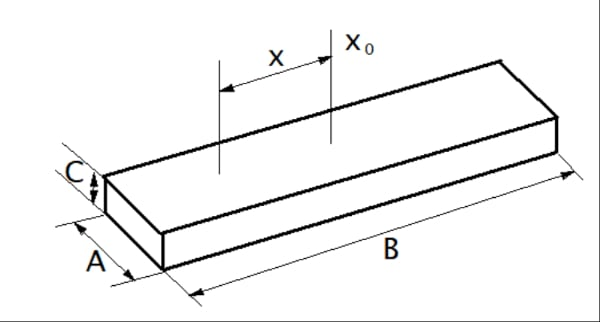##### Figure 5. Off-Centre Axis Moment of Inertia

The following equations are necessary for calculating the off-center moment of inertia:

$J_{x}=J_{x_{0}}+ml^{2}=\frac{1}{12}m(A^{2}+B^{2}+12l^{2})$

Where

Jx = inertia along x - axis

Jy = Inertia along y - axis

J$$_{x_{0}}$$ = Inertia along x0 - axis passing through the centre of gravity

m = mass

A = Width

B = Length

C = Height

ρ = Density

l = Distance between x and x0

#### Rectangular Pillar Moment of Inertia Calculation##### Figure 6. Rectangular pillar. Image used courtesy of Simon Mugo

The formula below will help solve the moment of inertia for the rectangular pillar above in Figure 6:

$J_{x}=\frac{1}{12}m\Big(A^{2}+B^{2}\Big)=\frac{1}{12}\rho ABC\Big(A^{2}+B^{2}\Big)$

And

$J_{y}=\frac{1}{12}m\Big(C^{2}+B^{2}\Big)=\frac{1}{12}\rho ABC\Big(C^{2}+B^{2}\Big)$

Where

Jx = inertia along x - axis

Jy = Inertia along y - axis

m = mass

A = Width

B = Length

C = Height

ρ = Density

#### Object in Linear Motion Moment of Inertia Calculation

$J=m(\frac{A}{2\pi})^{2}$

Where

A = Units of Moment

m = mass

#### Moment of Inertia Units of Measure

The units of inertia can be used in two ways: oz-in-sec2 and oz-in2. The former has an inclusion of mass while the latter involves gravity only.

Theoretically, the moment of inertia is a factor of mass only but, practically, inertia is affected by gravity; hence, one cannot ignore the effect of the earth’s gravity.

Gravity = 386 in/sec2

To convert oz-in2 to oz-in-sec2 take:

$\frac{oz-in^{2}}{386\,in/sec^{2}}=oz-in-sec^{2}$

### Determining the Torque Required

Torque is the ability of a force exerted on an object to make the object rotate. Torque is a combination of two elements: acceleration and load elements. Load torque is caused by friction. Acceleration torque is available only when the motor is in rotation. When the motor is running at a constant speed, the component disappears.

Torque is a product of force and the distance between this force and the center of rotation.

$T=Fr=\frac{FD}{2}$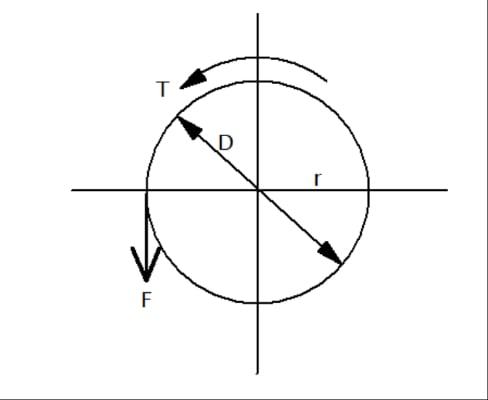#### Actual Measurement of the Load Torque

The most accurate force is obtained by measuring, since it improves efficiency and gives an accurate coefficient of friction.

FB = Force at the start of rotation of the main shaft:

$T_{L}=\frac{F_{B}D}{2}$

Where

D = Pulley final diameter and g = acceleration due to gravity

#### Force

Three types of force need to be determined: vertical, inclined, and horizontal.

#### Calculating Vertical Force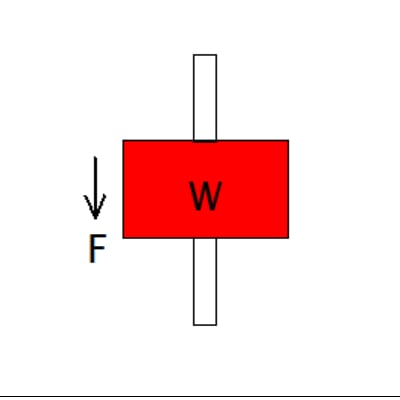F = W = mg

#### Calculating Horizontal Force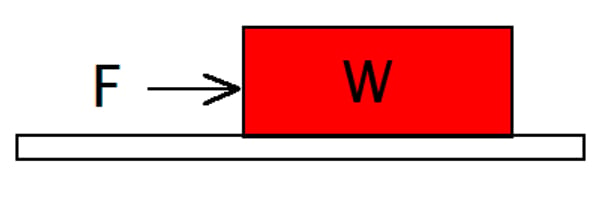F = μW

#### Calculating Inclined Force##### Figure 10. Inclined force. Image used courtesy of Simon Mugo

F = FA + mg(sinα + μ cosα)

Where

F = Moving direction force

μ = Friction coefficient of the sliding surface

g = gravity

m = mass of the table and load

FA = External Force

#### Ball Screw Driver Load Torque Calculation

$T_{L}=(\frac{FP_{B}}{2\pi\eta}+\frac{\mu_{0}F_{0}P_{B}}{2\pi})\frac{1}{i}$

$F=F_{A}+mg(sin\theta+\mu cos\theta)$

Where PB is the ball screw lead.

#### Pulley Drive Load Torque Calculation

$T_{L}=\frac{(\mu F_{A}+mg)D}{2i}$

#### Torque Calculation Equations for Belt or Wire Drive

$T_{L}=\frac{F}{2\pi\eta}\times\frac{\pi D}{i}=\frac{FD}{2\eta i}$

$F=F_{A}+mg(sin\theta+\mu cos\theta)$

Where

i = gear ratio

### Acceleration Torque

Acceleration torque is made up of acceleration rate and inertia. By knowing the two values, acceleration torque can be calculated.

Ta = JA

Where

Ta = Acceleration torque

A = Acceleration Rate

J = Inertia

### Speed

The speed of any particle is determined by measuring the distance it travels and dividing it by the time it takes to cover the distance.

For the stepper and servo motors, accounting for acceleration time is a must.

$Speed=\frac{Distance}{Time}$

Featured image used courtesy of Adobe Stock